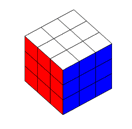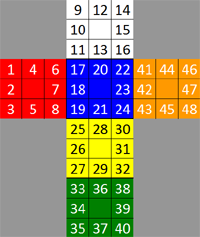# Problem 1139. Rubik's Cube: 30 Moves or Less : Contest Scoring (Time/Size/Moves)

This Challenge is to solve a thoroughly scrambled Rubik's cube with the best score (30 moves max). Score is Moves + Size/2000 + e^(avg time sec / 3).
Rubik's Cube can be solved in 20 moves or less from any position. The Kociemba Two Phase algorithm can solve the Cube in 30 moves or less. The basic theory is that any cube can transition to an H-cube by 12 moves using all possible 18 move types. An H-cube is defined as having no rotations or flips of corners or edges, respectively, and has all middle edges in the middle row. The cube can be solved from any H-cube state in 18 moves or less using only the moves U U' U2 D D' D2 F2 L2 R2 B2. Definitions of Moves / Rotates / Flips for Corners and Edges. The Two-Phase algorithm typically solves in 24 moves or less.Rubik's Cube has 48 faces that are assigned to a vector as in the above figure. U refers to the White face, F-Blue, L-Red, R-Orange, D-Yellow, and B-Green. Moves (1-6 UFDLBR 7-12 U'F'D'L'B'R' 13-18 U2F2D2L2B2R2) are clockwise looking towards the center, primes are CCW (eq U'), and twos are a half turn of a face.
The color to numeric coding is [RWBYGO] .
A solved cube is [0000 0000 1111 1111 2222 2222 3333 3333 4444 4444 5555 5555]
Input: Cube
Cube is a 48 long row vector of values 0 thru 5.
Output: Move_Vector
Move_Vector is an empty to 30 element vector of values 1:18
Constraint: Solution must be 30 moves or less
Scoring: Moves + Size / 2000 + e^(avg time sec / 3 )
Two Phase Source code is fairly complex and may require a 500MB database file. Its goal is to find solutions of 20 or less starting with Two Phase.

### Solution Stats

10.0% Correct | 90.0% Incorrect
Last Solution submitted on Jun 29, 2022

### Community Treasure Hunt

Find the treasures in MATLAB Central and discover how the community can help you!

Start Hunting!